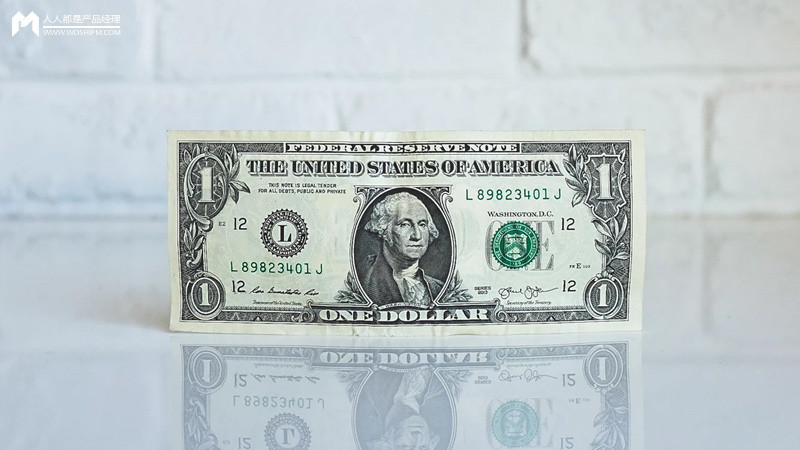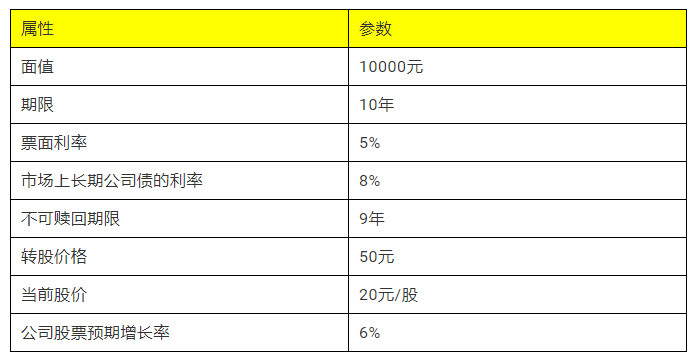APP## 金融产品经理之债券产品设计## 实战

### 2. 分析（1）分析我们设计的这款金融产品税前的融资成本。

=股价×转换比率

=20×(1+6%)^9×200

=6757.92（元）

=未来各期利息现值+到期本金现值

=(10000+10000×5%)/(1+8%)

=9722.22（元）

10000=10000×5%×(P/A,M,9)+6757.92×(P/F,M,9)

（M-6%)/(7%-6%)=(10000-7400.85)/(6933.48-7400.85)

（2）分析我们设计的这款产品票面利率是否合理。

10000=10000×R×(P/A,8%,9)+6757.92×(P/F,8%,9)

### 3. 结论

=10000×5%×(P/A,8%,10)+10000×(P/F,8%,10)

=500×6.7101+4631.93

=7986.98（元）

10000×10.6%×(P/A,8%,10)+10000×(P/F,8%,10)

=1060×6.7101+4631.93

=11744.62（元）

## 结语

1. 不要把张小龙这类人纳入到普通产品经理的范畴，我相信99%的所谓产品经理与张小龙在做着完全不同的工作。

回复
1. 再普通的产品经理，也要把目光和格局放的远一些。不要因为自己普通，而失去自己成长为张小龙的理想。

回复
2. 券商投行发债日常吧….

回复
1. 发债日常也会有一套标准的金融产品做支撑的。

回复
3. 这个算是产品业务设计，和普通的软件产品经理还是不一样😂

回复
1. 产品经理的工作范围很广，并不仅仅是写个软件产品的需求，只会写需求太基础了。

回复